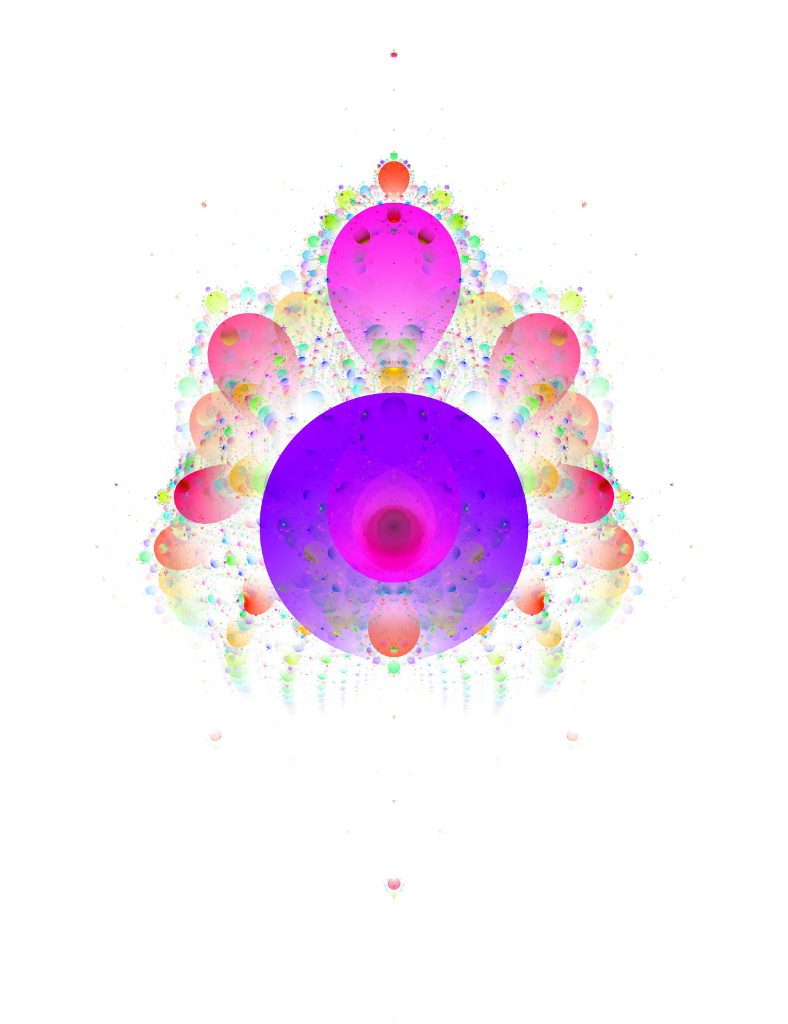# mathr

## Ultimate Anti-BuddhabrotThe Buddhabrot fractal is related to the Mandelbrot set: for each point $$c$$ that is not in the Mandelbrot set, plot all its iterates $$z_m$$ where $$z_0 = 0$$ and $$z_{n+1} = z_n^2 + c$$. The anti-Buddhabrot flips this, plotting all the iterates for points that are in the Mandelbrot set. For the Buddhabrot, the points not in the Mandelbrot set will escape to $$\infty$$, so we know when to stop iterating. Points in the interior don't escape, but almost all converge to a periodic cycle. In the limit of plotting a very large number of iterations, the iterates in these cycles will strongly dominate due to the periodic repetition, such that the earlier iterates will be invisible. Define the ultimate anti-Buddhabrot to be the iterate plots of these limit cycles.

Now, a point $$z_c$$ in the limit cycle for $$c$$ of period $$p$$ satisfies $$F^p(z_c, c) = z_c$$. There will be $$p$$ different $$z_c$$ for each $$c$$, but we just need one, and we can find it numerically using Newton's method. Given an arbitrary $$c$$ we need to find its period first, which we can do by checking the interior distance estimate for each partial. Define a partial as a $$q$$ such that $$|z_q| \lt |z_m|, 1 \le m \lt q$$. If the interior distance for $$c$$ is negative, then $$c$$ is not inside a component of the Mandelbrot set of that period. Once we have $$z_c$$, we can plot the $$p$$ iterates in the cycle.

Buddhabrot colouring commonly uses several monochrome image planes with different iteration limits, brighter where more iterates hit each pixel, which are combined into a colour image. With the ultimate anti-Buddhabrot, we can accumulate a colour image: each period is associated to an RGB value, and we can plot RGBA pixels (with A set to 1) with additive blending. The final A channel indicates how many iterates hit the pixel, but we also have the accumulated colours that can show which periods were involved. Post-processing the high dynamic range accumulation buffer to bring it down to something that can be displayed on a screen can bring out more of the details.

Calculation can be sped up using recursive subdivision. The root of the recursion passes over the Mandelbrot set parameter plane. There are a few different cases that can occur at each point:

exterior
Compute the exterior distance estimate: if it's so large that all of the subdivided child pixels would be exterior, bail out now, otherwise subdivide and recurse without plotting iterates.
interior
If the interior distance is so large that all of the subdivided child pixels would be interior to the same component, subdivide and recurse with the now-known period, otherwise recurse with the general method described above; plot the iterates in both cases.
unknown
If the iteration limit is reached, bail out without recursing.

The key speedup is switching to a simpler algorithm that just calculates $$z_c$$ and plots the iterates with a known period. Another optimisation exploits the symmetry of the Mandelbrot set about the real axis. Finally it's possible to parallelize using OpenMP, with atomic pragmas to avoid race conditions when accumulating pixels.

Source code in C99: Ultimate Anti-Buddhabrot reference implementation. Runtime just under 20mins on a 3GHz quadcore CPU.